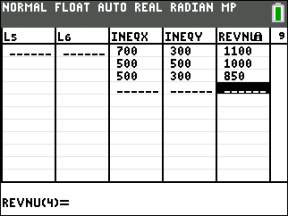##### Ti-84 Plus Graphing Calculator For Dummies, 2nd EditionLinear programming is a method for finding the maximum or minimum value of a multivariable function that is constrained by a system of inequalities. The following example should help you understand this rather technical definition of linear programming.

A chocolate company sells real and imitation chocolate chips to a local cookie factory. On any given day, the cookie factory needs at least 500 pounds of real chocolate chips and at least 300 pounds of imitation chocolate chips. The real chocolate chips sell for \$1.25 a pound and the imitation chocolate chips sell for \$0.75 a pound. If the truck that takes the chocolate chips to the cookie factory can carry at most 1,000 pounds of chocolate chips, how many pounds of each kind of chocolate chips should the chocolate company ship to the cookie factory in order to maximize its revenue?

In this example, the chocolate factory’s revenue is the function revenue = 1.25x + 0.75y, where x is the number of pounds of real chocolate chips and y is the number of pounds of imitation chocolate chips that the chocolate company ships to the cookie factory. The constraints stated in this example are: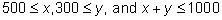In other words, this example asks you to find the maximum value of revenue = 1.25x + 0.75y subject to the system of constraints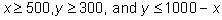How do you solve a linear programming problem? The following theorem gives the answer.

Linear Programming Theorem: If an optimum (maximum or minimum) value of a function constrained by a system of inequalities exists, then that optimum value occurs at one or more of the vertices of the region defined by the constraining system of inequalities.

This theorem tells you to evaluate the function at the points of intersection of the constraining system of inequalities. The smallest value found is the minimum value of the function and the largest is its maximum value. To get the Inequality app to help you solve a linear programming problem, follow these steps:

1. Graph the system of constraints.

The graph of the system of constraints appears in the third screen.

2. Graph the intersection of the regions in the graph.

The graph of the intersection appears in the second screen.

3. Find and store the points of intersection in the graph.

the process of finding the points of intersection is illustrated here.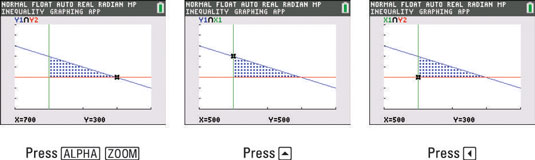4. Display the stored points of intersection.

The stored points of intersection appear here.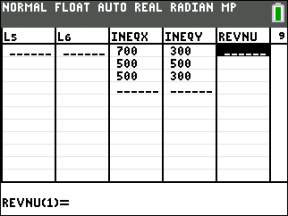5. Create a list to the right of list INEQY and give it a name.

The name you give the list should describe the function in the linear programming problem. In the next step, this function is evaluated at the stored points of intersection.

If an empty, unnamed list does not appear to the right of list INEQY, place the cursor in the heading of the third column and press [2nd][DEL] to insert a blank column, enter a name, and then press [ENTER]. If an empty, unnamed list does appear in the third column, place the cursor in the heading of that column, enter a name, and then press [ENTER].

6. Use a formula to define the entries in the new list.

The formula you enter is the formula that defines the function you want to optimize. In this example that formula is 1.25x + 0.75y, the definition of the revenue function. Because x is housed in list INEQX and y in INEQY, this formula is entered into the calculator as 1.25*∟INEQX + 0.75*∟INEQY.

To use a formula to define a list, place the cursor on the name of the list in the column heading. Because formulas should be surrounded by quotes, press [ALPHA][+] to enter the first quotation mark. Then enter the formula. To enter the name of a list, such as ∟INEQX, press [2nd][STAT] to display a list of the names of the lists in the Stat List editor.

Repeatedly press the down-arrow key to highlight the number to the left of the list and press [ENTER] to insert the name of the list in your formula. After entering the formula, press [ALPHA][+] to enter the closing quotation mark.

When you define a list, if you don’t use quotes around the formula, it will still generate a list. However, if you change the values in the list, other lists will not update accordingly. For that reason, it is a good idea to use quotes around a formula when defining a list.

7. Press e to evaluate the function at the points of intersection of the constraining system of inequalities.

According to the Linear Programming Theorem, if the function has a maximum and/or minimum value, those values appear in the list you just created. As illustrated here, the chocolate factory in the example can maximize its revenue by shipping 700 pounds of real chocolate chips and 300 pounds of imitation chocolate chips.